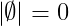# How to denote empty set symbol(∅) in LaTeX?

Empty set is a unique set in which no element is stored. Empty set is also called null set. Mathematically, the Empty set is denoted by the Phi ø and curly brackets {}.

 Properties Value Symbol Empty set Argument No Commands \emptyset \O Example \emptyset →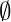However, the use of this {} symbol is very rare in the case of Empty sets. In most cases, these ø symbol are used. Latex has more than one command to denote both symbols.

## O-Slash(ø) Symbol in LaTeX

Method 1. In latex, the \emptyset command is commonly used for the O-Slash symbol. For example

\documentclass{article}
\begin{document}
$p=\left \{ x:x^{2}=4,x\:is\:odd\right \}$
$\therefore p=\emptyset$
\end{document}

Output :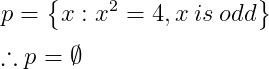Notice the latex program above, the \: command has been used to free up space between two words in the equation.

Method 2. Second, you can use the \O command instead of the \emptyset command for the same symbol. The meaning of the symbol is the same but it is slightly different in structure.

\documentclass{article}
\begin{document}
$p=\left \{ x:x^{2}=9,x\:is\:even\right \}$
$\therefore p=\O$
\end{document}

Output :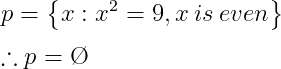Looking at the output, you can see that the \therefore command has been used for the symbol.

Method 3. Third, I will use the \varnothing command, but for this command you must first define the amssymb package.

As you probably all know,\usepackage commands are used when defining a package. And the package has to be passed in the command as an argument.

\documentclass{article}
\usepackage{amssymb}
\begin{document}
$$p=\left \{ x:x\:is\:odd\right$$
$$q=\left \{ x:x\:is\:even\right$$
$$\therefore p\cap q=\varnothing$$
\end{document}

Output :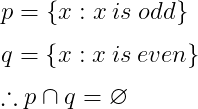The above three methods are represented in front of you. In my opinion, the first method would be the best practice for empty set symbols. For which there is no need to define any package. And in most mathematical documents, the \emptyset command is used for the Empty set symbol.

## Curly bracket symbol in latex for empty set

If you look at your computer keyboard, you will see that Carly bracket is present. You can use direct curly bracket in latex for the Empty set.

But, I will tell you to use \left \{  \right \} command instead of using direct curly bracket. As a result, the size of the curly bracket symbol will dynamically decrease and increase according to the text.

\documentclass{article}
\begin{document}
$p=\left \{ \right \}$
\end{document}

Output :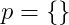## Value of empty set in LaTeX

The value of the Empty set is always zero. Thus, the value of the empty set is represented by the absolute value symbol.

\documentclass{article}
\begin{document}
$\left | \emptyset \right |=0$
\end{document}


Output :